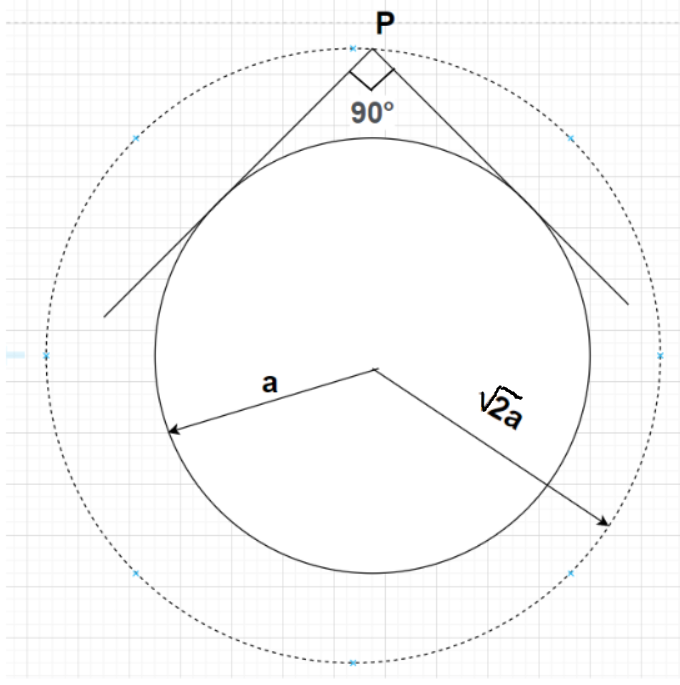Courses
Courses for Kids
Free study material
Free LIVE classes
MoreLIVE
Join Vedantu’s FREE Mastercalss

# Find the equation of the director circle of the circle ${\left( {x - h} \right)^2} + {\left( {y - k} \right)^2} = {a^2}$Verified
362.4k+ views
Hint- Here, we will be using the general equation of the director circle to any given circle. The required equation of the director circle is obtained by finding the radius of the given circle and then multiplying it with $\sqrt 2$ in order to get the radius of the director circle.

Given equation of circle is ${\left( {x - h} \right)^2} + {\left( {y - k} \right)^2} = {a^2}{\text{ }} \to {\text{(1)}}$

The general equation of any circle having centre coordinates (b,c) and radius r is given by
${\left( {x - b} \right)^2} + {\left( {y - c} \right)^2} = {r^2}{\text{ }} \to {\text{(2)}}$
By comparing equations (1) and (2), we have
The centre coordinates of the given circle is (h,k) and radius of the given circle is a.
As we know that the director circle is the locus of the point of intersection of two perpendicular tangents to the given circle.

For any general circle whose equation is ${\left( {x - b} \right)^2} + {\left( {y - c} \right)^2} = {r^2}$, the equation of director circle is given by ${\left( {x - b} \right)^2} + {\left( {y - c} \right)^2} = {\left( {\sqrt 2 r} \right)^2}$.

Clearly, in the case of a director circle the coordinates of the centre of the director circle and the given circle will be the same whereas the radius of the director circle will become $\sqrt 2$ times the radius of the given circle.

So, the radius of the director circle for the given circle ${\left( {x - h} \right)^2} + {\left( {y - k} \right)^2} = {a^2}$ whose radius is a will become $\sqrt 2 a$ and the centre coordinates of the director circle being the same as that of the i.e., (h,k).
So, the equation of director circle with centre coordinates (h,k) and radius $\sqrt 2 a$ is given by
${\left( {x - h} \right)^2} + {\left( {y - k} \right)^2} = {\left( {\sqrt 2 a} \right)^2} \\ \Rightarrow {\left( {x - h} \right)^2} + {\left( {y - k} \right)^2} = 2{a^2} \\$
Hence equation of the director circle of the circle ${\left( {x - h} \right)^2} + {\left( {y - k} \right)^2} = {a^2}$ is ${\left( {x - h} \right)^2} + {\left( {y - k} \right)^2} = 2{a^2}$.

Note- In this problem, the figure is drawn and point P in the figure represents the point of intersection of two perpendicular tangents to the given radius. The locus of this point P will give the director circle to the given circle (any point on the outer circle whose radius is $\sqrt 2 a$ corresponds to the point of intersection of two perpendicular tangents to the smaller circle whose radius is a).

Last updated date: 22nd Sep 2023
Total views: 362.4k
Views today: 7.62k